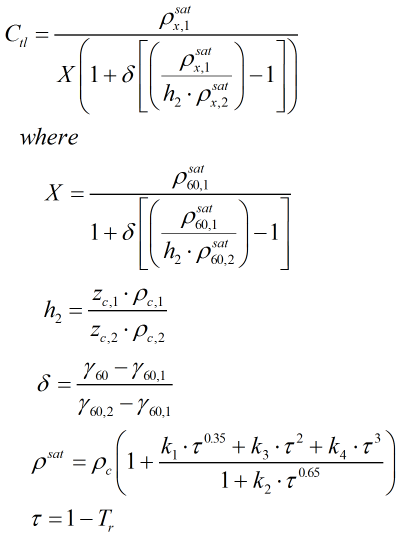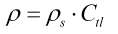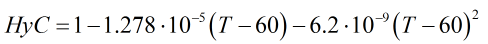# C293 – GPA TP-27:2007 – Temperature Correction for NGL and LPG

## Description

This calculates the temperature correction factor for NGL and LPG’s. This can then be used to obtain a density at standard or line conditions.

GPA TP-27/API MPMS Chapter 11.2.4 – Temperature Correction for the Volume of NGL and LPG Tables 23E, 24E, 53E, 54E, 59E and 60E (2007)

Kelton calculation reference C293

FLOCALC calculation reference F090

KIMS calculation reference K228

## Options

### Standard Temperature

• 15°C
• 20°C
• 60°F

This option is used to select the standard temperature which the liquid is to be referred against.

### Solve for

• Density
• Standard density

This option used to select whether the density the user wishes to solve for is the line or standard density.

### Precision

• Full precision
• Full rounding
• Rounding of inputs only

This option is used to decide upon the rounding procedure to be employed. The full precision calculation doesn’t round any values. Full rounding rounds the inputs and outputs as described in the standard. Rounding of inputs only rounds only the input temperature and density according to the procedure set out in the standard. Note that the range of the density inputs at standard conditions vary from one standard temperature to another. Consult the standard for details of the range of the input parameters of the calculation. Inputs that do not meet the criteria described will output NaN as a result.

### Density Input

• Density
• Relative density

If 60°F is selected as standard temperature, i.e Table 23E or 24E is being employed, there will be the option to enter the liquid density as a relative density.

### Correct for

• Hydrometer

If the options for 60°F standard temperature, to solve for standard density and to enter a relative density are selected an option to apply a hydrometer correction will become available. This only needs to be selected if a glass hydrometer was used obtain the relative density value so as to correct for the expansion/contraction of the glass.

### Constants

Density of water at 60°F ρH2O_60°F = 999.016 kg/m³

## Calculation

### Temperature Correction

The Ctl is calculated by using an iterative process unless the standard temperature is 60°F. The iterative regime converts the standard density to the relative density at 60°F. The calculation works by referencing this relative density value against the properties of 2 reference fluids at a standard temperature of 60°F. An algorithm determines which reference fluids should be used according to the relative density of the input fluid. A further algorithm then calculates the Ctl from a series of relationships stemming from the reference fluid parameters. The temperature correction factor, Ctl, is calculated by:Where ρsat = Saturation density ρc = Critical density zc = Critical compressibility factor γ60 = Relative density at 60°F Tr = Reduced temperature k = Saturation density fitting parameters

### Density

Density is calculated from the relationship:Where ρs = Standard density

### Hydrometer Correction

The hydrometer correction for relative densities measured with a glass hydrometer is as follows:Where T = Temperature in °F

Back